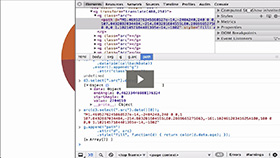Basic Chart - Donut Chart
Duration: 18:46
Part of Course: Introductory D3 Course
This Lesson is for subscribersUnlock this lesson NOW!
• Get expert advice on Basic Chart - Donut Chart
• Start mastering D3.js right now!

Takeaways:

• Notice that the code is exactly the same as the pie chart other than how the D3 Arc Generator Function innerRadius is defined
• Notice that this chart uses the same pattern regarding with nested data - first create an object and then create a secondary nested object inside of the first object for each data object in the data array
• You will use the CSV data from the D3js.org website Pie Chart example to see how a full D3 Pie Chart example data visualization is built
• Notice that the styling is done in the <style> </style> section of the HTML document
• Notice the D3 Margin Convention
• Notice the D3 Ordinal Scale to be used for colors
• Notice the D3 SVG Arc Path Generator Function and its accessor functions
• Notice that the innerRadius function is based on a formula rather than a constant like in the Basic Chart - Pie Chart example
• Notice the D3 Pie Chart Layout Helper
• Notice how the data is passed into the D3 Pie Chart Layout helper which then gets passed into the D3 Data Operator
• Notice the D3 Data Join
• Notice the SVG paths being appended to the SVG Group elements (and inherinting the bound data)
• Notice the D3 SVG Arc Path Generator Function being used to set the "d" attribute of the SVG Path
• Notice the use of the D3 SVG Arc Path Generator function centroid method being used to lable the donut chart slices

This Transcript is for subscribers.Unlock this D3 Tutorial NOW!
• Get expert advice on Basic Chart - Donut Chart